# Get and Set DDP's in Groovy Script(Part-2)

## This blog demonstrates how to Get and Set DDP's In Groovy ScriptStep 11:

Now, we will be able to see the command prompt as shown in the screenshot.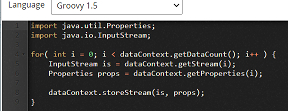Step 12:

Now, we are writing a groovy script to get DDP’s which are set in set properties shape and add the values which we have provided as static. The result will be saved in new Dynamic Document Property named as “sum” which is generated.

NOTE:  DDP refers to Dynamic Document Property in this blog.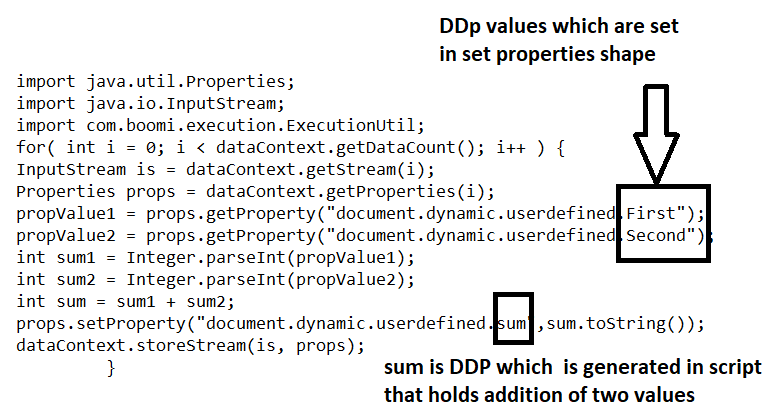• In the below 2 steps, we get Dynamic Document Properties (i.e. First and Second) which we have set in set properties shape by using props.getProperty() method.

propValue1 = props.getProperty("document.dynamic.userdefined.First");

propValue2 = props.getProperty("document.dynamic.userdefined.Second");

• The DDP’s that are retrieved from set properties shape are stored in propValue1 and propValue2.

We are using Integer.parseInt() methodto convert the values coming from propValue1 and propValue2 into integer and stores it in sum1 and sum2  reference variables.

int sum1 = Integer.parseInt(propValue1);

int sum2 = Integer.parseInt(propValue2);

int sum = sum1 + sum2;

• We can set the Dynamic document property by using the method props.setProperty() which takes2 parameters. The first parameter would be the key named as “sum” and the second parameter would be the result of sum1 and sum2 values which are coming from the above step.

props.setProperty("document.dynamic.userdefined.sum",sum.toString())

• Add the script in command prompt and click Ok.

Step 13:

Now, Drag and drop message shape onto process canvas.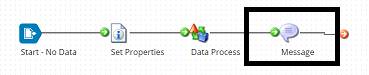• Select the shape and in message, give it as Value is {1}. Here {1} would be the runtime value which comes DDPsum.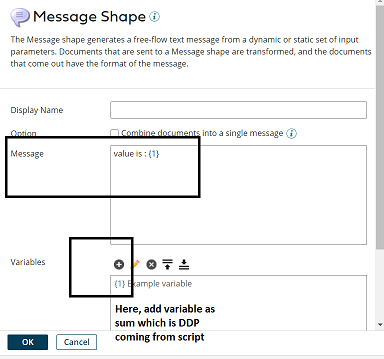• To configure the variable as DDP sum, click on + and select Type as Document Property and click choose and select Dynamic Document Property from drop down.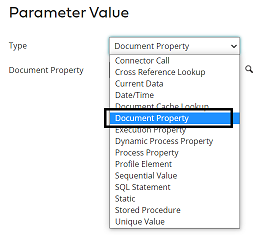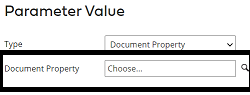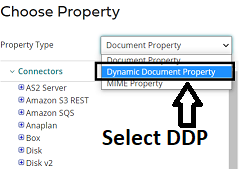• Now, give the property name as sum and click ok.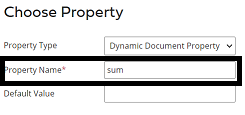• Now, we see that {1} is configured with DDP which is sum generated in script and holds the result. Click Ok.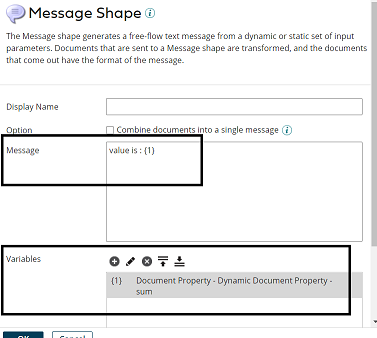Step 14:

Drag and drop the stop shape after message shape to indicate the end.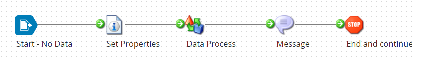Step 15:

Now, test the process by selecting the atom.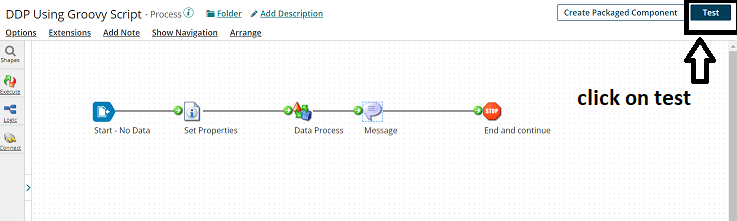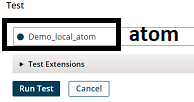• We see that the process havebeen executed successfully and have received the output from DDP i.e. sum.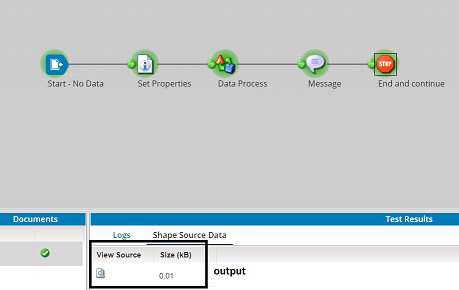• Click on view source and we will be able to see the output.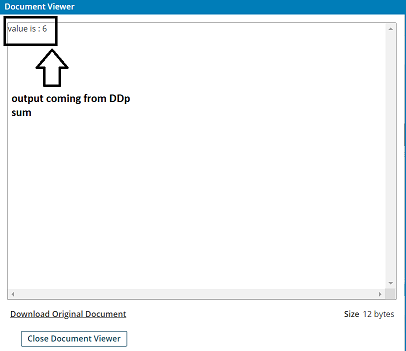#### What's Your Reaction?0000000